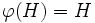# Subnormal automorphism-invariant subgroup

## Contents

BEWARE! This term is nonstandard and is being used locally within the wiki. [SHOW MORE]
This article defines a subgroup property: a property that can be evaluated to true/false given a group and a subgroup thereof, invariant under subgroup equivalence. View a complete list of subgroup properties[SHOW MORE]

## Definition

A subgroup$H$ of a group$G$ is termed a subnormal automorphism-invariant subgroup if, given any automorphism$\varphi$ of$G$ such that$\varphi$ restricts to an automorphism for every subnormal subgroup of$G$,$\varphi(H) = H$.

## Formalisms

### In terms of the invariance-closure operator

This property is obtained by applying the invariance-closure operator to the property: subnormal subgroup
View other properties obtained by applying the invariance-closure operator